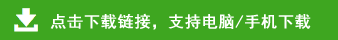# 新人教版一年级上册数学期末复习达标测试卷有答案

新人教版一年级上册数学期末复习达标测试卷有答案部分内容预览

一、计算题。（33分）
1、口算。（30分）
5+7=    14+4=    5-4=    18-8=    15+4=
15-10=    6-3=    7+7=    16-2=    11+7=
2+8=    4+9=    19-10=    4+7=    8+8=
10+9=    12+1=    18-15=    19-1=    4+4=
16-1=    9+7=    4-1=    3+8=    14-2=
18+1=    12-2=    11+6=    10-2=    2+2=
3-2=    18-3=    10+4=    17-3=    9-3=
8-3=    15+1=    2+9=    8+5=    9-4=
4+2=    8-4=    12+2=    4-4=    5+2=
19-5=    15+2=    10-5=    7-5=    0+5＝
9+9=    19-6=    0+9=    10-6=    9-6=
4+1=    6-6=    18-7=    9+6=    8-7=
2、直接写出得数。（3分）
3+7-5=                  2+6-3=           12-4-4=
8+5-3=                  9-4+6=           2+3+5=
二、填空题。（30分）
1、看图写数。
2、在最多的下面画△，最少的画√。
3、请把方格里缺少的数填上。
4、在○里填上“＞”“＜”或“＝”。
5○9              13○12            18○9             20○16
11＋4○12         15○8＋9　4＋7○11          8＋8○17
5、想一想，填一填。
（1）17里面有(    )个一和(    )个十。2个十是（    ）。
（2）12前面的数是（    ），17后面的数是（    ）。
（3）一个加数是5，另一个加数是9，和是（     ）
（4）13–3=10中，被减数是（     ），减数是（     ），差是（     ）。
（5）
一共有（    ）个小动物。
小鹿排第一，小熊排第（    ），小兔排第（    ）。
从左边数，请把第4个小动物圈起来。
6、从6、7、8、15四个数中选出三个数,列出两道加法算式和两道减法算式。
□○□=□    □○□=□
□○□=□    □○□=□
7、把下面的算式按得数从小到大排一排。
7+2     10–3      5+6      8–4      3+3
﹤      ﹤       ﹤      ﹤